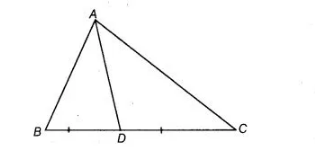# AD is a median of the ΔABC.`
Question:

Solution:

Yes,In $\triangle A B D$, we have $A B+B D>A D$$\ldots($ i)

[sum of any two sides of a triangle is greater than third side]

In $\triangle A C D$, we have $A C+C D>A D$ ....(ii)

[sum of any two sides of a triangle is greater than third side]

On adding Eqs. (i) and (ii), we get

$(A B+B D+A C+C D)>2 A D$

$\Rightarrow \quad(A B+B D+C D+A C)>2 A D$

Hence, $A B+B C+A C>2 A D$ $[\because B C=B D+C D]$